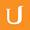## Binomial Multiplied by a Trinomial - Visualizing Algebra

• 0:00 - 0:04
In the case of multiplying a binomial by a trinomial, we just want to add some
• 0:04 - 0:08
rectangles so we can complete our multiplication. Just like with these numbers,
• 0:08 - 0:12
I'm going to set up a rectangle, and then I'm going to label each factor along
• 0:12 - 0:16
the side of the rectangle. This one will be x plus 3, and this one will be x
• 0:16 - 0:20
squared plus 2x plus 3. Using what you know about multiplication, I want you to
• 0:20 - 0:24
figure out the area of each of these rectangles. What would they be?
Title:
Binomial Multiplied by a Trinomial - Visualizing Algebra
Video Language:
English
Team:Udacity
Project:
MA006 - Visualizing Algebra
Duration:
0:25Udacity Robot edited English subtitles for Binomial Multiplied by a Trinomial - Visualizing AlgebraCogi-Admin added a translation

# English subtitles

## Revisions Compare revisions

• API
Udacity Robot